# An Evaluation Algorithm for the Interoperability of Global Navigation Satellite Systems

An Evaluation Algorithm for the Interoperability of Global Navigation Satellite Systems
College of Automation, Harbin Engineering University, Harbin 150001, China

College of Information and Communication engineering, Harbin Engineering University, Harbin 150001, China

Institute of Deep-sea Science and Engineering, Chinese Academy of Sciences, Sanya 572000, China

Corresponding Author Email:
cxb@hrbeu.edu.cn
Page:
137-144
|
DOI:
https://doi.org/10.18280/ts.370118
8 June 2019
|
Accepted:
17 November 2019
|
Published:
29 February 2020
| Citation

OPEN ACCESS

Abstract:

The interoperability of global navigation satellite systems (GNSSs) has a significant impact on the service performance of GNSSs. To evaluate the GNSS interoperability, this paper creates an evaluation algorithm with such modules as space section, user section and environment section. The proposed algorithm evaluates the service performance with several common parameters, namely, the dilution of precision (DOP), navigation satellite system precision (NSSP), navigation satellite system integrity (NSSI), availability and continuity. The parameters of the algorithm could be configured based on the existing GNSS data or our self-developed data. Then, the proposed algorithm was applied to evaluate the service performance of three difference scenarios: Compass-only, Compass + GPS and Compass + GPS + Galileo. The results show that the combination of different GNSSs can greatly improve the service performance of GNSSs in both time and space.

Keywords:

global navigation satellite systems (GNSSs), Compass/BeiDou Navigation Satellite System (Compass), interoperability, evaluation, service performance

1. Introduction

Global navigation satellite systems (GNSSs) refer to satellite systems that pinpoint the geographic location of a user's receiver anywhere in the world. Several GNSSs are currently in operation, namely, the United States’ Global Positioning System (GPS), and Russia’s GLObal NAvigation Satellite System (GLONASS). The number of GNSS satellites is expected to reach 120 or more by 2020. The performance of the GNSSs is improved by augmentation systems like the European Geostationary Navigation Overlay Service (EGNOS). Better performance could be achieved if the navigation signals of different GNSS are combined into a solution with high accuracy and availability. The capability for user equipment to produce such a combined solution is called GNSS interoperability.

The GNSS interoperability has been widely studied in terms of reference frame, constellation selection, receiver performance, etc. Píriz et al.  analyzed the GPS to Galileo time offset (GGTO) and timing biases of the interoperability between GPS and Galileo In-Orbit Validation Element (GIOVE). Zhang et al.  introduced the signal-in-space reception to monitor the time offset of GNSS at the time scale of National Time Service Center (NTSC). Forrest  explored the interoperability of the GPS and Galileo timescales for positioning and metrology, and explained the implications of positioning receivers with various time offsets for a mixed constellation in urban environment. Focusing on constellation orbit selection, Fan et al.  proposed two optimized interoperability schemes for GLONASS and Galileo, and selected an interoperability scheme for Compass and Galileo at an altitude of 24,126km. Li et al.  found that the losses of some satellites may differ slightly in dilution of precision (DOP) but could impose a negative impact on integrity performance, and presented a novel weighting algorithm to select the optimal set of satellites that features high accuracy and good integrity.

The signal design of GNSSs has also attracted much attention from the academia. Yao et al.  developed an evaluation method for signal performance under the mode of generic mismatch processing, put forward four reference modulations for interoperable signal of Compass B1 band, and selected the reference modulation with the best overall performance. Amin  examined the effects of jitter on GPS and Galileo navigation signals, and suggested modelling jitter effects as an additive noise to limit the achievable signal-to-noise ratio (SNR) under sinusoidal input signals. Floch et al.  designed a flexible and systematic design for GNSS signals, and applied the design to a potential new service in S-band. Based on three signal combinations, Boulanger et al.  took commercial-off-the-shelf (COTS) receivers as data recorder, and computed position, velocity and time (PVT) on real datasets in advanced configurations.

The latest modernized GNSSs provide users with various navigation services and signals. Kovar et al.  implemented a self-designed E-L correlator to the field programmable gated array (FPGA) of GNSS receiver software, and tested the correlator on Galileo E1b, E1c, and E5a signals. Peres et al.  described the architecture and features of the REAGE receiver, and validated the receiver’s performance on GPS L1 and Galileo E1 signals. Odijk and Teunissen  constructed the mixed GPS + Galileo model based on inter-system double differencing, i.e. differencing the Galileo phase and code observations relative to those corresponding to the reference or pivot satellite of GPS.

It is a great challenge to evaluate interoperability as a key metric of GNSS performance. The existing evaluation algorithms mainly focus on the receiver level and the system level. Han et al.  introduced an interoperability evaluation algorithm based on the differential equation dynamical system, and verified the significance of the algorithm through example analysis. Zhang et al.  illustrated the hardware environment for the operation of evaluation codes, and discovered that the evaluation results vary with condition attributes. Wang et al.  put forward an innovative assessment algorithm for GNSS interoperability at the system level, and demonstrated that interoperability can greatly improve GNSS performance.

Galileo System Simulation Facility (GSSF) and Satellite Tool Kit (STK) are popular tools to provide the simulation environment of GNSS interoperability. Kalden et al.  reported that the Galileo System Simulation Facility (GSSF) can reproduce the functions and performance of Galileo, and enjoys the in-built flexibility to support system simulation for the construction of Galileo. Zimmermann et al.  introduced the raw data generation (RDG) capability of the GSSF, and identified potential applications of this capability within Galileo. Zhang et al.  determined the maximum service range of reference receiver for mobile positioning in Assisted Global Navigation Satellite System (AGNSS), and created a simulation environment for the GNSS spatial constellation and ground facilities based on the STK. Fazliani et al.  carried out a coverage analysis with the aid of the STK. However, the GSSF and STK are so well packaged that their parameters cannot be adjusted flexibly to suit the needs of the research into the GNSS interoperability.

Therefore, this paper attempts to design a flexible algorithm that effectively evaluates various GNSS interoperability schemes, namely, Compass + GPS and Compass + GPS + Galileo, and simulate the evaluation algorithm on MATLAB. The parameters of the algorithm could be configured based on the existing GNSS data or our self-developed data.

2. Preliminaries

Let A be a matrix, $A^{T}$ be the transpose of matrix A, and $A^{-1}$ be the inverse of matrix A. The background knowledge for our algorithm is introduced below.

2.1 DOP

The distance between the i-th satellite to user $\rho_{i}$ can be derived easily from the position $\left(T_{x i}, T_{y i}, T_{z i}\right)$ of the i-th satellite and the position $\left(R_{x}, R_{y}, R_{z}\right)$ of the user in the earth-centered, earth-fixed (ECEF) coordinate system.

The user position in three dimensions can be determined according to the measurements of four satellites:

$\rho_{i}=f\left(R_{x}, R_{y}, R_{z}, t_{u}\right)$

$=\sqrt{\left(T_{x i}-R_{x}\right)^{2}+\left(T_{y i}-R_{y}\right)^{2}+\left(T_{z i}-R_{z}\right)^{2}}+c t_{u}$    (1)

where, i falls in [1, 4]; c is the speed of sound underwater; $t_{u}$ is the offset of the receiver clock from system time.

Let (x,y,z) be the approximate position of the user. Then, the deviation of the true position from the approximate position can be denoted as (Δx,Δy,Δz), and the time bias estimate as u. On this basis, the following equations can be derived:

$R_{x}=x+\Delta x$

$R_{y}=y+\Delta x$

$R_{z}=z+\Delta x$

$t_{u}=u+\Delta u$    (2)

Then, we have:

$f\left( {{R}_{x}},{{R}_{y}},{{R}_{z}},{{t}_{u}} \right)=f\left( x+\Delta x,y+\Delta y,z+\Delta z,u+\Delta u \right)$    (3)

Based on the approximate position of the user, formula (3) can be rewritten by Taylor series expansion as:

\begin{align} & f\left( x+\Delta x,y+\Delta y,z+\Delta z,u+\Delta u \right)=f\left( x,y,z,u \right)+ \\ & +\frac{\partial f\left( x,y,z,u \right)}{\partial x}\Delta x+\frac{\partial f\left( x,y,z,u \right)}{\partial y}\Delta y+\frac{\partial f\left( x,y,z,u \right)}{\partial z} \\ & \Delta z+\frac{\partial f\left( x,y,z,u \right)}{\partial u}\Delta u\cdots \\ \end{align}    (4)

To eliminate nonlinear terms, the expansion was truncated after the first-order partial derivatives:

$\begin{matrix} \frac{\partial f\left( x,y,z,u \right)}{\partial x}=-\frac{{{T}_{xi}}-x}{{{d}_{i}}} \\ \frac{\partial f\left( x,y,z,u \right)}{\partial y}=-\frac{{{T}_{yi}}-y}{{{d}_{i}}} \\ \frac{\partial f\left( x,y,z,u \right)}{\partial z}=-\frac{{{T}_{zi}}-z}{{{d}_{i}}} \\ \frac{\partial f\left( x,y,z,u \right)}{\partial u}=c \\\end{matrix}$    (5)

where,

${{d}_{i}}=\sqrt{{{\left( {{T}_{xi}}-x \right)}^{2}}+{{\left( {{T}_{yi}}-y \right)}^{2}}+{{\left( {{T}_{zi}}-z \right)}^{2}}}$    (6)

Then, we have:

${{\rho }_{i}}={{d}_{i}}-\frac{{{T}_{xi}}-x}{{{d}_{i}}}\Delta x-\frac{{{T}_{yi}}-y}{{{d}_{i}}}\Delta y-\frac{{{T}_{zi}}-z}{{{d}_{i}}}\Delta z+c\Delta$    (7)

For convenience, four new variables are introduced below:

$\begin{matrix} \Delta {{d}_{i}}={{d}_{i}}-{{\rho }_{i}} \\ {{D}_{xi}}=\frac{{{T}_{xi}}-x}{~{{d}_{i}}} \\ {{D}_{yi}}=\frac{{{T}_{yi}}-y}{~{{d}_{i}}} \\ {{D}_{zi}}=\frac{{{T}_{zi}}-z}{~{{d}_{i}}} \\\end{matrix}$    (8)

By now, there are four unknown quantities: $(\Delta x, \Delta y, \Delta z)$ and Δu, which can be solved based on measurements from four satellites. These quantities can be determined by solving the following set of linear equations:

$\Delta d=H\Delta X$    (9)

where, Δd and H are defined as:

$\Delta \mathbf{d}=\left[\begin{array}{c}\Delta d_{1} \\ \Delta d_{2} \\ \Delta d_{3} \\ \Delta d_{4}\end{array}\right] \mathbf{H}=\left[\begin{array}{cccc}D_{x 1} & D_{y 1} & D_{z 1} & 1 \\ D_{x 2} & D_{y 2} & D_{z 2} & 1 \\ D_{x 3} & D_{y 3} & D_{z 3} & 1 \\ D_{x 4} & D_{y 4} & D_{z 4} & 1\end{array}\right] \Delta \mathbf{X}=\left[\begin{array}{c}\Delta x \\ \Delta y \\ \Delta z \\ \Delta u\end{array}\right]$    (10)

Formula (10) can be solved by the least squares (LS) method:

$\Delta X={{\left( {{H}^{\text{T}}}H \right)}^{-1}}{{H}^{\text{T}}}\Delta d$    (11)

The above evaluation scheme works only if the displacement is close to the actual point. The allowable displacement can be determined based on the accuracy requirement on the receiver. If the displacement exceeds the acceptable value, the $d_{i}$ should be replaced with a new measurement estimated based on (x,y,z).

The covariance of ΔX can be derived from $\Delta X \cdot \Delta X^{T}$ and an expectation:

\begin{aligned} \operatorname{cov}(\Delta \mathbf{X})=\mathrm{E}\left[\Delta \mathbf{X} \cdot \Delta \mathbf{X}^{\mathrm{T}}\right] &=\mathrm{E}\left[\left(\mathbf{H}^{\mathrm{T}} \mathbf{H}\right)^{-1} \mathbf{H}^{\mathrm{T}} \Delta \mathbf{d} \cdot\left(\left(\mathbf{H}^{\mathrm{T}} \mathbf{H}\right)^{-1} \mathbf{H}^{\mathrm{T}} \Delta \mathbf{d}\right)^{\mathrm{T}}\right] \\ &=\mathrm{E}\left[\left(\mathbf{H}^{\mathrm{T}} \mathbf{H}\right)^{-1} \mathbf{H}^{\mathrm{T}} \Delta \mathbf{d} \cdot \Delta \mathbf{d}^{\mathrm{T}} \mathbf{H}\left(\mathbf{H}^{\mathrm{T}} \mathbf{H}\right)^{-1}\right] \\ &=\left(\mathbf{H}^{\mathrm{T}} \mathbf{H}\right)^{-1} \mathbf{H}^{\mathrm{T}} \operatorname{cov}(\Delta \mathbf{d}) \mathbf{H}\left(\mathbf{H}^{\mathrm{T}} \mathbf{H}\right)^{-1} \end{aligned}    (12)

It is generally assumed that $\omega_{i}$ obeys an independent Gaussian distribution with zero mean and a variance of $\sigma^{2}$. Under this assumption, the covariance of Δd is a scalar multiple of the n´n identity matrix $I_{n \times n}$:

$\text{cov}\left( \Delta d \right)={{I}_{\text{n}\times \text{n}}}{{\sigma }^{2}}$    (13)

Substituting formula (13) to formula (12), we have:

$\text{cov}\left( \Delta X \right)={{\left( {{H}^{\text{T}}}H \right)}^{-1}}{{H}^{\text{T}}}H{{\left( {{H}^{\text{T}}}H \right)}^{-1}}{{\sigma }^{2}}={{\left( {{H}^{\text{T}}}H \right)}^{-1}}{{\sigma }^{2}}$    (14)

Under the above assumption, the covariance of position errors is a scalar multiple of the matrix $\left(H^{T} H\right)^{-1}$:

$\left(\mathbf{H}^{\mathrm{T}} \mathbf{H}\right)^{-1}=\left[\begin{array}{cccc}\mathrm{P}_{1,1} & \mathrm{P}_{1,2} & \mathrm{P}_{1,3} & \mathrm{P}_{1,4} \\ \mathrm{P}_{2,1} & \mathrm{P}_{2,2} & \mathrm{P}_{2,3} & \mathrm{P}_{2,4} \\ \mathrm{P}_{3,1} & \mathrm{P}_{3,2} & \mathrm{P}_{3,3} & \mathrm{P}_{3,4} \\ \mathrm{P}_{4,1} & \mathrm{P}_{4,2} & \mathrm{P}_{4,3} & \mathrm{P}_{4,4}\end{array}\right]$    (15)

Then, the most general parameter is named the geometric dilution of precision (GDOP) :

$\text{GDOP}=\sqrt{{{P}_{1,1}}+{{P}_{2,2}}+{{P}_{3,3}}+{{P}_{4,4}}}$    (16)

To evaluate the component accuracy of position/time parameters, several other DOP parameters in common applications are of great use: position dilution of precision (PDOP), horizontal dilution of precision (HDOP), vertical dilution of precision (VDOP), and time dilution of precision (TDOP):

$\text{PDOP}=\sqrt{{{P}_{1,1}}+{{P}_{2,2}}+{{P}_{3,3}}}$    (17)

$\text{HDOP}=\sqrt{{{P}_{1,1}}+{{P}_{2,2}}}$    (18)

$\text{VDOP}=\sqrt{{{P}_{3,3}}}$    (19)

$\text{TDOP}=\sqrt{{{P}_{4,4}}}$    (20)

Of course, the difference between GNSSs in geodetic and time coordinate reference model must be considered in the computing process.

2.2 Navigation satellite system precision (NSSP)

The NSSP is generally defined as:

$\sigma _{i}^{2}={{\left( \sigma _{BC}^{2}+\sigma _{BE}^{2}+\sigma _{iono}^{2}+\sigma _{tropo}^{2}+\sigma _{rec}^{2}+\sigma _{multipath}^{2} \right)}_{i}}$    (21)

where, $\sigma_{i}^{2}$ is the variance of user equivalent range error (UERE); $\sigma_{\mathrm{BC}}^{2}, \sigma_{\mathrm{BE}}^{2}, \sigma_{i o n o}^{2}, \sigma_{\mathrm{tropo}}^{2}, \sigma_{\mathrm{rec}}^{2}$ and $\sigma_{\text {multipath }}^{2}$ are corrections induced by broadcast clock, broadcast ephemeris, ionosphere delay, troposphere delay, receiver noise and multipath, respectively.

Let $\left(\mathrm{H}^{\mathrm{T}} \mathrm{WH}\right)^{-1}$ be matrix Q and W be a diagonal matrix whose diagonal components are $\sigma_{i}^{-2}$. Then, the five NSSP parameters, namely, global NSSP (GNSSP), position NSSP (PNSSP), horizontal NSSP (HNSSP), vertical NSSP (VNSSP) and time NSSP (TNSSP), can be respectively computed by:

$\text{GNSSP}=\sqrt{{{Q}_{1,1}}+{{Q}_{2,2}}+{{Q}_{3,3}}+{{Q}_{4,4}}}$    (22)

$\text{PNSSP}=\sqrt{{{Q}_{1,1}}+{{Q}_{2,2}}+{{Q}_{3,3}}}$    (23)

$\text{HNSSP}=\sqrt{{{Q}_{1,1}}+{{Q}_{2,2}}}$    (24)

$\text{VNSSP}=\sqrt{{{Q}_{3,3}}}$    (25)

$\text{TNSSP}=\sqrt{{{Q}_{4,4}}}$    (26)

2.3 Navigation satellite system integrity (NSSI)

The NSSI provides prompt warnings to users once the system is in error. The NSSI value can be captured by Receiver Autonomous Integrity Monitor (RAIM). The RAIM outputs the horizontal protection level (HPL):

$HPL=SLOP{{E}_{\max }}\times pbias$    (27)

where, $\mathrm{SLOPE}_{\max }$ is the maximum slope relative to visible satellites. Let $I_{n}$ be an n×n unit matrix. Then, the slope relative to the i-th satellite can be obtained by:

$B=P{{A}^{\text{T}}}$    (28)

$S={{I}_{n}}-AB$    (29)

$SLOPE\left( i \right)=\sqrt{B_{1,i}^{2}+B_{2,i}^{2}}/\sqrt{{{S}_{ii}}}$    (30)

In addition, pbias is the critical bias in parity space, which depends on the number of visible satellites :

$pbias={{\sigma }_{i}}\sqrt{\lambda }$    (31)

where, λ is the non-centrality of the non-central chi-square density function.

2.4 Availability

Availability stands for the ratio of time that DOP, NSSP or HPL is below the threshold to the navigation duration. The availability in the period of [tstart, tend] can be defined as:

Availability$=\frac{\sum_{t=t_{\text {start}}}^{t_{\text {end}}} \text {Evaluation }\{\text {value}(t) \leq \text {thrashold}\}}{1+\left(t_{\text {end}}-t_{\text {start}}\right) / T}$    (32)

where, value(t) is the value of DOP, NSSP or HPL over the simulation duration; if value(t) is smaller than or equal to threshold, the Evaluation{value(t)≤thrashold} is 1; otherwise, the Evaluation{value(t)≤thrashold} is zero; T is the sample time interval.

2.5 Continuity

Continuity describes the probability that the GNSS performs stably in the navigation duration. The continuity in the period $t_{t o p}$ of $\left[t, t+t_{t o p}\right]$ can be determined as:

$Continuity=\frac{\sum\nolimits_{t={{t}_{start}},inc=T}^{{{t}_{end}}-{{T}_{op}}}{\prod\nolimits_{u=t,inc=T}^{t+{{T}_{op}}}{\left( Evaluation\left\{ value\left( t \right)\le thrashold \right\} \right)}}}{\sum\nolimits_{t={{t}_{start}},inc=T}^{{{t}_{end}}-{{T}_{op}}}{Evaluation\left\{ value\left( t \right)\le thrashold \right\}}}$    (33)

3. Algorithm Design

## 1.jpg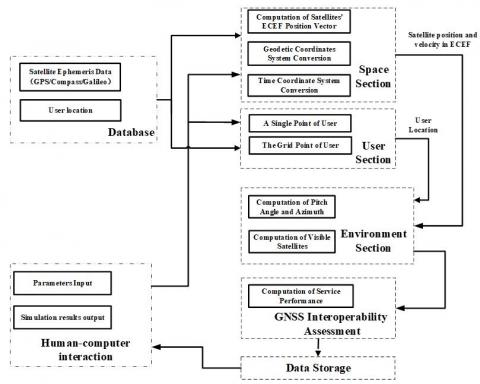Figure 1. Our GNSS interoperability evaluation algorith

As shown in Figure 1, our GNSS interoperability evaluation algorithm consists of seven parts. The seven GNSS ephemeris data are provided in the database. The satellite’s position and velocity in ECEF are computed with the GNSS ephemeris data defined in the database of the space section, according to Kaplan and Christopher’s formula (2015). The GNSS ephemeris data involve the seven common parameters of the GNSS ephemeris message, namely, time of epoch and the osculating Keplerian elements at the time of epoch. Nine other parameters are provided to correct the Keplerian elements as functions of time after epoch. Moreover, the conversions between reference coordinate systems for geodetic and time are provided. The choices of the constellations, including the number of satellites, are defined in the human-computer interaction (HCI) section.

The user location is defined in the user section, with two optional modes. In the one mode, the user location is defined as a single point with longitude and latitude. In the other mode, the user location is described as grid points with an interval of longitude and latitude, and meanwhile, the resolution is taken into consideration. The user location can be selected either through the database or the HCI. The conversion of user location from geodetic coordinates to Cartesian coordinates in the ECEF frame is specified by Kaplan and Christopher (2005).

The satellite’s position and velocity, plus the user location, are inputted to the environment section. The output from this section includes the pitch angle and azimuth from the satellites to the user. The satellites are visible to the user when the pitch angle is greater than the mask degree. In the GNSS interoperability module, the DOP, NSSP and NSSI are calculated with the information from the environment section. The availability and continuity are solved in the next section.

The simulation data ae saved in the format of MATLAB, text and database in the data storage section. The evaluation data are analyzed in the HCI module. Many simulation parameters are obtained from this module for simulation, including the start and end time of the simulation, the time step, the combination of constellations, number of satellites, mask angle, user location, probability of missed detection and false alarm, and the threshold of GNSS interoperability.

4. Simulation and Results Analysis

Our algorithm was developed on the MATLAB, which features concise programming, abundant function resources and an outstanding graphical user interface (GUI). The software offers multiple tools to analyze the GNSS interoperability. Figure 2 shows the HCI of our algorithm. Note that 1:33 means all the 33 satellites of the Compass system are adopted for this simulation; the east longitude and south latitude are expressed as negative numbers. For example, “-180” and “-90” means 180°E and 90°S, respectively.

## 2.jpg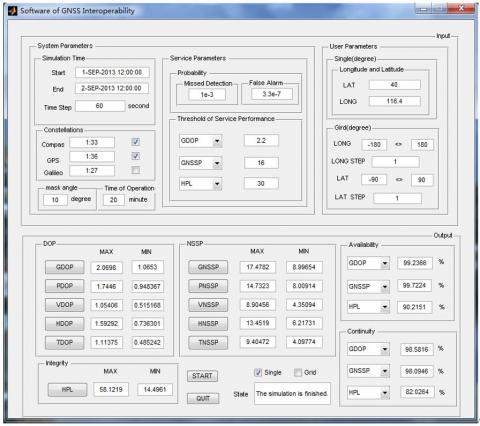Figure 2. The HCI of our algorithm

In the Compass, 3 satellites operate on geostationary orbit (GEO), 24 on medium earth orbit (MEO) and 3 on inclined geosynchronous orbit (IGSO). In the GPS, 36 satellites are distributed on 6 orbital planes. In the Galileo, 27 satellites are distributed on 3 orbital planes, in a so-called Walker 27/3/1 constellation.

During the simulation, the mask angle was set to 10°; the probabilities of false alarm and missed detection were set to  $3.3 \times 10^{-7}$ and $1 \times 10^{-3}$, separately.

4.1 Single-point simulation

The single-point simulation was carried out for 24h at an observation station in Beijing $\left(40^{\circ} N, 116.4^{\circ} E\right)$, with the time step of 60s. The service performance can be measured by the DOP, availability and continuity. The greater the values of these indices, the better the service performance will be. Figures 3-8 display the simulation results on DOP parameters and HPL of three scenarios: the Compass-only scenario, the Compass + GPS scenario, and the Compass + GPS + Galileo scenario; Table 1 lists the statistics on NSSP of the three scenarios; Figures 9 and 10 exhibit the availability and continuity of GNSSP, respectively, of the three scenarios.

## 3.jpg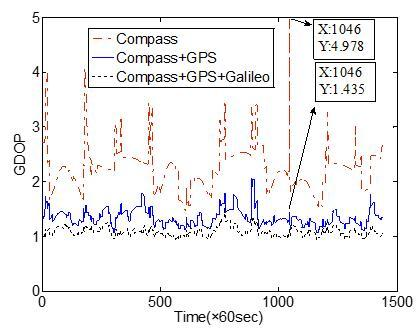Figure 3. Time variations in GDOP

## 4.jpg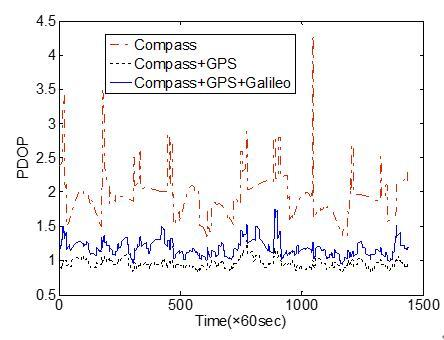Figure 4. Time variations in PDOP

## 5.jpg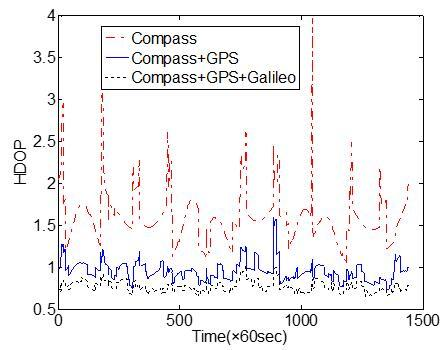Figure 5. Time variations in HDOP

## 6.jpg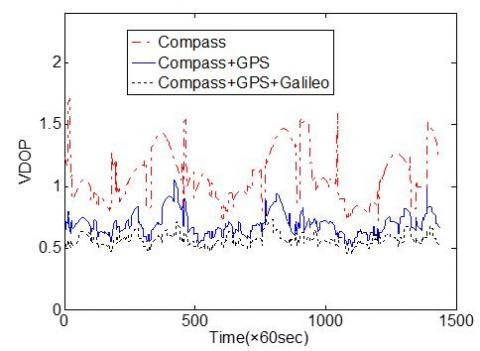Figure 6. Time variations in VDOP

## 7.jpgFigure 7. Time variations in TDOP

## 8.jpg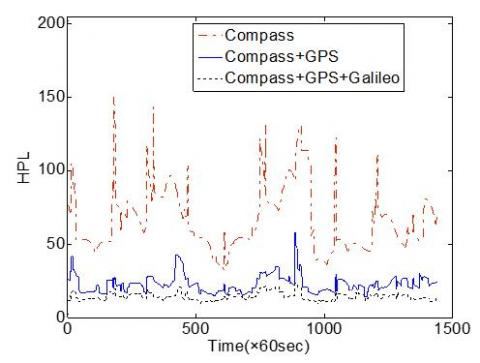Figure 8. Time variations in HPL

As shown in Figures 3-8, the Compass + GPS scenario outperformed the Compass-only scenario in all DOP parameters and the HPL, but was outshined by the Compass + GPS + Galileo scenario, at any sampling point. A natural reason is that the more the GNSSs, the greater the number of visible satellites, and the better the combined performance. In addition, the DOP parameters and HPL of the Compass + GPS scenario were far greater than those of the Compass-only scenario. For example, the GDOP at 1046×60s of the Compass-only scenario was 4.978, while that of the Compass + GPS scenario was 1.435.

Table 1. Statistics on the NSSP

 GNSS feature GNSSP PNSSP HNSSP VNSSP TNSSP Compass The best 12.12 10.93 8.931 5.490 5.236 The worst 23.16 19.33 15.70 11.54 12.74 The span 11.03 8.401 6.774 6.054 7.513 Compass+GPS The best 9.527 8.607 7.263 4.197 4.083 The worst 16.60 14.17 12.74 9.595 8.786 The span 7.077 5.562 5.483 5.398 4.703 Compass+GPS+Galileo The best 8.073 7.221 6.090 3.586 3.594 The worst 12.13 10.43 9.381 6.074 6.195 The span 4.065 3.217 3.291 2.487 2.601 NSSP improvement of two system The best 21.39% 21.25% 18.56% 23.42% 21.97% The worst 54.07% 47.21% 33.04% 35.59% 75.60% The span 32.68% 25.96% 14.48% 12.01% 53.79% NSSP improvement of three system The best 33.44% 34.00% 31.72% 34.66% 31.34% The worst 90.94% 81.37% 70.83% 99.84% 125.20% The span 57.49% 47.44% 39.015% 65.02% 94.02%

As shown in Table 1, the peak NSSP of the Compass + GPS scenario was 20% higher than that of the Compass-only scenario, but 32% lower than that of the Compass + GPS + Galileo scenario. Thus, the more the GNSSs, the better the NSSP value will be. The improving effect of the number of GNSSs was even more prominent on the minimum NSSP.

## 9.jpgFigure 9. The availability of GNSSP

## 10.jpg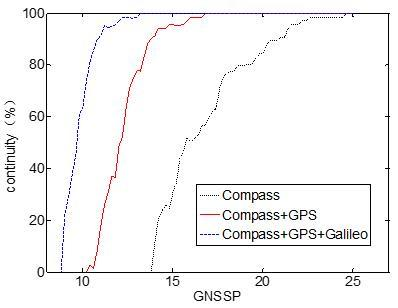Figure 10. The continuity of GNSSP

The maximum allowable GNSSP depends on the desired accuracy. As shown in Figures 9 and 10, the availability and continuity of the GNSSP varied with the thresholds. The steepest curves of availability and continuity belong to the Compass + GPS + Galileo scenario, the second steepest curves belong to the Compass + GPS scenario, and the least steep curves belong to the Compass-only scenario. Hence, the combined system can greatly improve the availability and continuity of GNSSP.

To sum up, the single-point simulation shows that the GNSS interoperability can compensate for the coverage of satellites, and enhance the service performance over time.

4.2 Grid point simulation

The service performance of three scenarios was simulated again over global grid points of 180 degree of three scenarios, with the latitude range of 90°, the longitudes range of 180°, the resolution of 1°×1°. The simulation lasted 24h with the time step of 600s. The mean GDOP at a point in space at different time points was computed, and then the mean GDOP of the entire earth was plotted (Figures 11-13).

## 11.jpgFigure 11. Mean GDOP of Compass-only scenario

## 12.jpg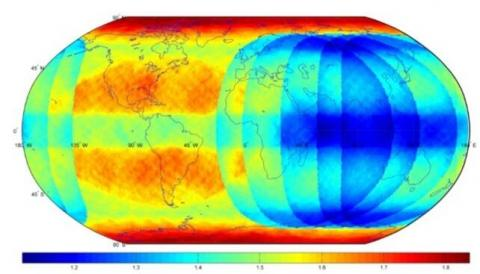Figure 12. Mean GDOP of Compass + GPS scenario

## 13.jpgFigure 13. Mean GDOP of Compass + GPS + Galileo scenario

5. Conclusions

This paper develops an evaluation algorithm for GNSS interoperability on the MATLAB. The DOP, NSSP, NSSI, availability and continuity were obtained through the algorithm, and used to evaluate the service performance of GNSSs. The parameters of the algorithm could be configured based on the existing GNSS data or our self-developed data.

To verify its performance, the proposed algorithm was applied to evaluate the time- and space-based performance of three difference scenarios: Compass-only, Compass + GPS and Compass + GPS + Galileo. The results show that the GNSS interoperability can greatly enhance the service performance in both time and space.

Acknowledgements

This work was supported by National Natural Science Foundation of China (61633008, 61374007) and Natural Science Foundation of Heilongjiang Province (F2017005).

References

 Píriz, R., García, Á.M., Tobías, G., Fernández, V., Tavella, P., Sesia, I., Hahn, J. (2008). GNSS interoperability: Offset between reference time scales and timing biases. Metrologia, 45(6): 87-102. https://doi.org/10.1088/0026-1394/45/6/S14

 Zhang, H., Zhu, L., Li, X., Jiang, H., Zhang, X. (2014). GNSS system time offset monitoring at NTSC. 2014 IEEE International Frequency Control Symposium (FCS), pp. 1-5. https://doi.org/10.1109/FCS.2014.6859957

 Forrest, W.M. (2004). Interoperability of the GPS and Galileo timescales for positioning and metrology. 18th European Frequency and Time Forum, EFTF 2004, pp. 468-475. https://doi.org/10.1049/cp:20040912

 Fan, L., Yang, X., Jiang, C., Wang, Z. (2012). Constellation orbits selection considering the interoperability between different GNSS. International Academy of Astronautics Conference on Dynamics and Control of Space Systems, Porto. 145: 1365-1373.

 Li, L., Yuan, H., Yuan, C., Wei, D., Liu, W. (2013). GNSS Satellite Selection Algorithm Revisited: A Weighted Way with Integrity Consideration. 4th China Satellite Navigation Conference, CSNC 2013, pp. 173-187. https://doi.org/10.1007/978-3-642-37404-3_16

 Yao, Z., Liu, L., Zhang, X., Lu, M. (2012). GNSS interoperable signal modulation for multiple receiving modes. Geomatics and Information Science of Wuhan University, 37(5): 621-625.

 Amin, B. (2007). Jitter Analysis of QPSK and BOC (n, n) GNSS Signals. 20th International Technical Meeting of the Satellite Division of The Institute of Navigation 2007, pp. 1543-1548.

 Floch, J.J., Antreich, F., Meurer, M., Issler, J.L. (2010). S-Band Signal Design Considering Interoperability and Spectral Separation. 23rd International Technical Meeting of the Satellite Division of the Institute of Navigation 2010, pp. 3349-3358.

 Boulanger, C., Bonhoure, B., Suard, N., Lapeyre, D. (2011). New open GNSS signals: First combined GPS/GLONASS/GIOVE experiments. Proceedings of the 24th International Technical Meeting of The Satellite Division of the Institute of Navigation, pp. 2821-2828.

 Kovar, P., Kacmarik, P., Vejrazka, F. (2011). Interoperable GPS, GLONASS and Galileo software receiver. IEEE Aerospace and Electronic Systems Magazine, 26(4): 24-30. https://doi.org/10.1109/MAES.2011.5763340

 Peres, T.R., Silva, J.S., Silva, P.F., Carona, D., Serrador, A., Palhinha, F., Véstias, M. (2014). Multi-GNSS receiver for aerospace navigation and positioning applications. International Archives of the Photogrammetry. Remote Sensing and Spatial Information Sciences - ISPRS Archives, 87-92. https://doi.org/10.5194/isprsarchives-XL-3-W1-87-2014

 Odijk, D., Teunissen, P.J. (2013). Characterization of between-receiver GPS-Galileo inter-system biases and their effect on mixed ambiguity resolution. GPS Solutions, 17(4): 521-533. https://doi.org/10.1007/s10291-012-0298-0

 Han, T., Lu, X., Wang, X., Rao, Y., Zou, D., Yang, J., Wu, Y. (2011). Differential equation dynamical system based assessment model in GNSS interoperability. Science China, 54(6): 996-1003. https://doi.org/10.1007/s11433-011-4337-6

 Zhang, S., Ju, T., Ren, C. (2012). An Evaluation of Multi-GNSS Receiver’s Interoperability. 3rd China Satellite Navigation Conference, CSNC 2012, pp. 125-132. https://doi.org/10.1007/978-3-642-29175-3_12

 Wang, W., Lv, C.C., Li, X. (2014). Assessment Models and Rules of GNSS Interoperability. Advanced Materials Researchm. 2014: 808-811. https://doi.org/10.4028/www.scientific.net/AMR.846-847.808

 Kalden, O., Schmidt-Brücken, F., Zimmermann, F., Pfister, J. (2012). Galileo System Simulation Facility recent applications & Developments. 2012 6th ESA Workshop on Satellite Navigation Technologies (Navitec 2012) & European Workshop on GNSS Signals and Signal Processing, pp. 1-6. https://doi.org/10.1109/NAVITEC.2012.6423085

 Zimmerman, F., Haak, T., Steindl, E., Vardarajulu, S., Kalden, O., Hill, C. (2005). Generating Galileo raw data-approach and application. Proceedings of DASIA 2005 - Data Systems in Aerospace, 602: 487-490.

 Zhang, G.H., Meng, W.X., He, Y., Ren, J.Q. (2010). Research on the Service Range of Reference Receiver in AGNSS Mobile Positioning. 2010 WRI International Conference on Communications and Mobile Computing. https://doi.org/500-504. 10.1109/CMC.2010.275

 Fazliani, Y., Unwin, M., Jales, P. (2012). Satellite-borne remote sensing: Using space GPS/GNSS receiver for reflectometry. 6th ESA Workshop on Satellite Navigation Technologies: Multi-GNSS Navigation Technologies Galileo's Here. NAVITEC 2012 and European Workshop on GNSS Signals and Signal Processing, pp. 1-4. https://doi.org/10.1109/NAVITEC.2012.6423102

 Kaplan, E. D. (2005). Understanding GPS: Principles and Applications. Second Edition, Artech House, Norwood.

 Brown, R.G., Chin, G.Y. (1988). GPS RAIM: Calculation of thresholds and protection radius using Chi-Square Methods-A geometric approach. Navigation ION Red Book Series, Radio Technical Commission for Aeronautics.